# Vedic maths- its relevance to real learning

26 Oct 2017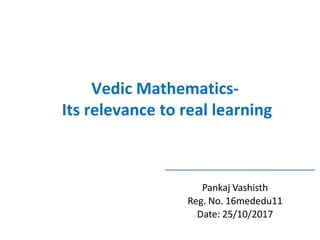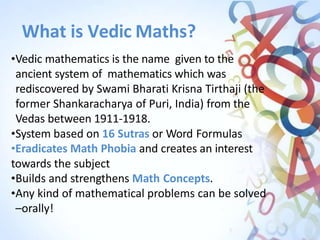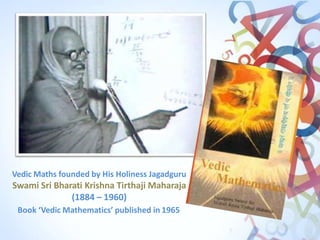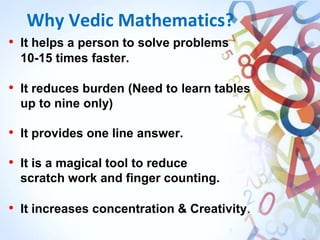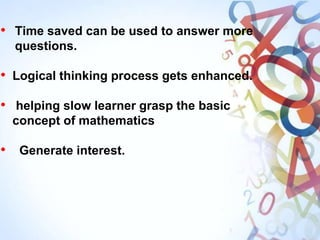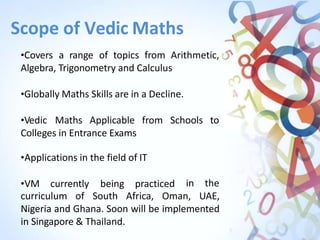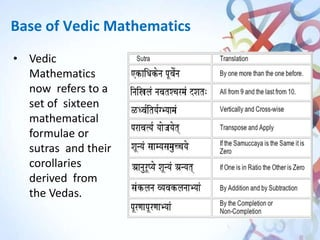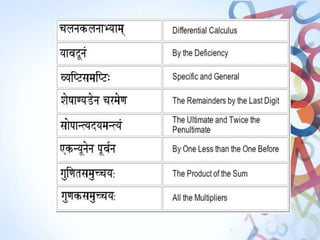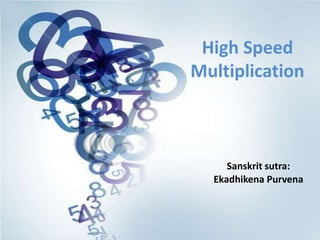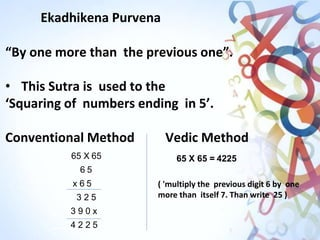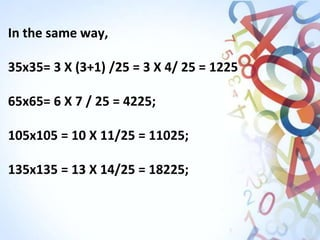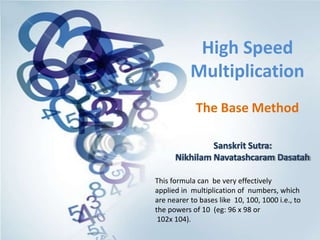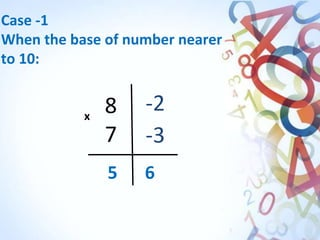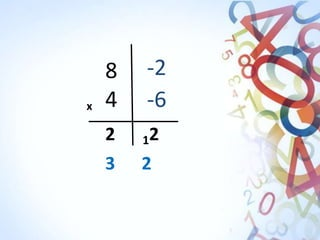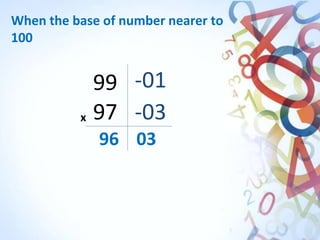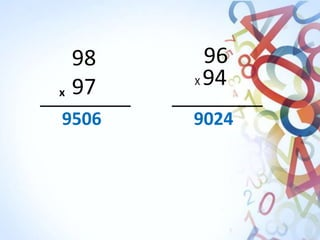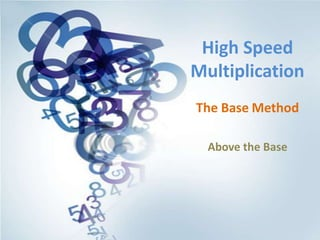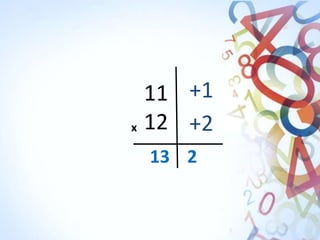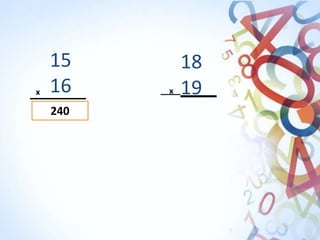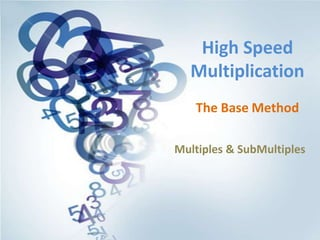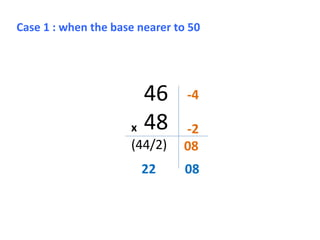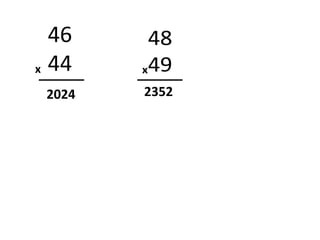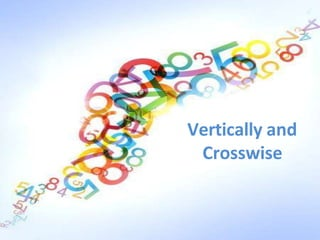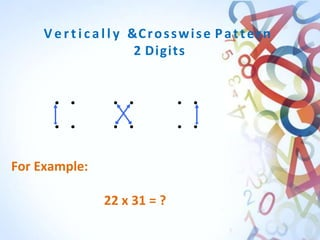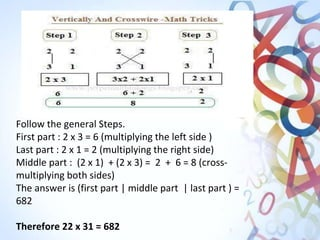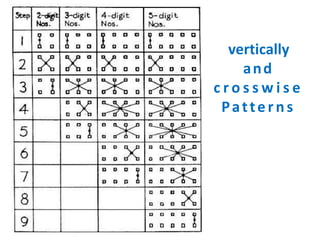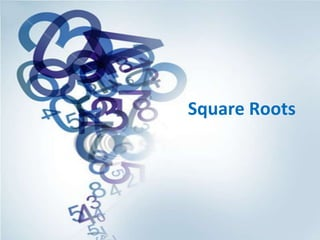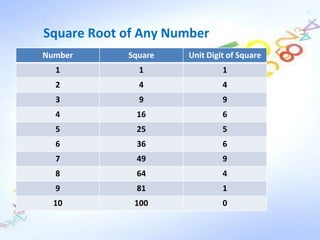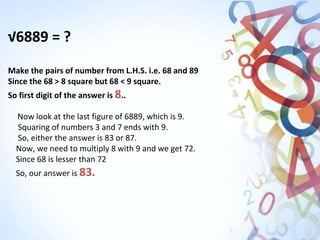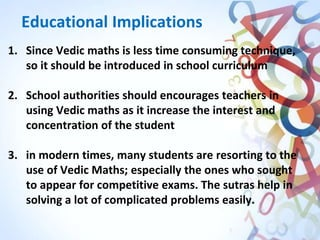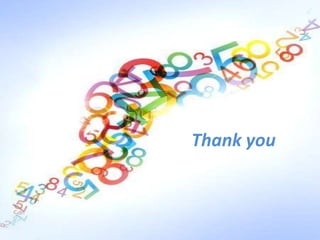1 sur 31

### Vedic maths- its relevance to real learning

• 1. Pankaj Vashisth Reg. No. 16mededu11 Date: 25/10/2017 Vedic Mathematics- Its relevance to real learning
• 2. What is Vedic Maths? •Vedic mathematics is the name given to the ancient system of mathematics which was rediscovered by Swami Bharati Krisna Tirthaji (the former Shankaracharya of Puri, India) from the Vedas between 1911-1918. •System based on 16 Sutras or Word Formulas •Eradicates Math Phobia and creates an interest towards the subject •Builds and strengthens Math Concepts. •Any kind of mathematical problems can be solved –orally!
• 3. Vedic Maths founded by His Holiness Jagadguru Swami Sri Bharati Krishna Tirthaji Maharaja (1884 – 1960) Book ‘Vedic Mathematics’ published in 1965
• 4. Why Vedic Mathematics? • It helps a person to solve problems 10-15 times faster. • It reduces burden (Need to learn tables up to nine only) • It provides one line answer. • It is a magical tool to reduce scratch work and finger counting. • It increases concentration & Creativity.
• 5. • Time saved can be used to answer more questions. • Logical thinking process gets enhanced. • helping slow learner grasp the basic concept of mathematics • Generate interest.
• 6. Scope of Vedic Maths •Covers a range of topics from Arithmetic, Algebra, Trigonometry and Calculus •Globally Maths Skills are in a Decline. •Vedic Maths Applicable from Schools to Colleges in Entrance Exams •Applications in the field of IT •VM currently being practiced in the curriculum of South Africa, Oman, UAE, Nigeria and Ghana. Soon will be implemented in Singapore & Thailand.
• 7. Base of Vedic Mathematics • Vedic Mathematics now refers to a set of sixteen mathematical formulae or sutras and their corollaries derived from the Vedas.
• 10. Ekadhikena Purvena “By one more than the previous one”. • This Sutra is used to the ‘Squaring of numbers ending in 5’. Conventional Method Vedic Method 65 X 65 6 5 x 6 5 3 2 5 3 9 0 x 4 2 2 5 65 X 65 = 4225 ( 'multiply the previous digit 6 by one more than itself 7. Than write 25 )
• 11. In the same way, 35x35= 3 X (3+1) /25 = 3 X 4/ 25 = 1225 65x65= 6 X 7 / 25 = 4225; 105x105 = 10 X 11/25 = 11025; 135x135 = 13 X 14/25 = 18225;
• 12. High Speed Multiplication The Base Method Sanskrit Sutra: Nikhilam Navatashcaram Dasatah This formula can be very effectively applied in multiplication of numbers, which are nearer to bases like 10, 100, 1000 i.e., to the powers of 10 (eg: 96 x 98 or 102x 104).
• 13. 8 7 -2 -3 5 6 x Case -1 When the base of number nearer to 10:
• 15. 96 03 99 -01 x 97 -03 When the base of number nearer to 100
• 17. High Speed Multiplication The Base Method Above the Base
• 20. High Speed Multiplication The Base Method Multiples & SubMultiples
• 21. 46 x 48 (44/2) Case 1 : when the base nearer to 50 22 08 -4 -2 08
• 24. V e r t i c a l l y &Crosswise Pattern 2 Digits For Example: 22 x 31 = ?
• 25. Follow the general Steps. First part : 2 x 3 = 6 (multiplying the left side ) Last part : 2 x 1 = 2 (multiplying the right side) Middle part : (2 x 1) + (2 x 3) = 2 + 6 = 8 (cross- multiplying both sides) The answer is (first part | middle part | last part ) = 682 Therefore 22 x 31 = 682
• 26. vertically and c r o s s w i s e Patterns
• 28. Square Root of Any Number Number Square Unit Digit of Square 1 1 1 2 4 4 3 9 9 4 16 6 5 25 5 6 36 6 7 49 9 8 64 4 9 81 1 10 100 0
• 29. √6889 = ? Make the pairs of number from L.H.S. i.e. 68 and 89 Since the 68 > 8 square but 68 < 9 square. So first digit of the answer is 8.. Now look at the last figure of 6889, which is 9. Squaring of numbers 3 and 7 ends with 9. So, either the answer is 83 or 87. Now, we need to multiply 8 with 9 and we get 72. Since 68 is lesser than 72 So, our answer is 83.
• 30. Educational Implications 1. Since Vedic maths is less time consuming technique, so it should be introduced in school curriculum 2. School authorities should encourages teachers in using Vedic maths as it increase the interest and concentration of the student 3. in modern times, many students are resorting to the use of Vedic Maths; especially the ones who sought to appear for competitive exams. The sutras help in solving a lot of complicated problems easily.